0%

# 绪论（二）

## 思维导图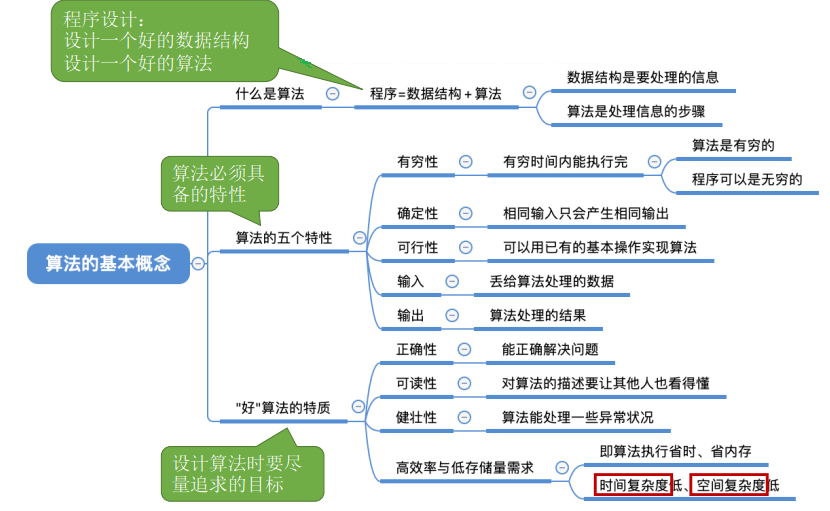## 1.3 算法

### 1.3.1 算法的特性

1. 有穷性。一个算法必须总是在执行有穷步后结束，且每一步都必须在有穷时间内完成。
2. 确定性。对千每种情况下所应执行的操作，在算法中都有确切的规定，不会产生二义性， 使算法的执行者或阅读者都能明确其含义及如何执行。
3. 可行性。算法中的所有操作都可以通过已经实现的基本操作运算执行有限次来实现。
4. 输入。一个算法有零个或多个输入。当用函数描述算法时，输入往往是通过形参表示的， 在它们被调用时，从主调函数获得输入值。
5. 输出。一个算法有一个或多个输出，它们是算法进行信息加工后得到的结果，无输出的 算法没有任何意义。当用函数描述算法时，输出多用返回值或引用类型的形参表示

1. 正确性。算法应能够正确地解决求解问题。
2. 可读性。算法应具有良好的可读性，以帮助人们理解。
3. 健壮性。输入非法数据时，算法能适当地做出反应或进行处理，而不会产生莫名其妙的输出结果。
4. 高效率与低存储量需求。花的时间少。时间复杂度低，不费内存。空间复杂度低

### 1.3.2 时间复杂度（1）加法规则：多项相加，只保留最高阶的项，且系数变为1

$T(n) = T_1(n) + T_2(n) = O(f(n)) + O(g(n)) = O(max(f(n), g(n)))$

（2）乘法规则：多项相乘，都保留

$T(n) = T_1(n)×T_2(n) = O(f(n))×O(g(n)) = O(f(n)×g(n))$

$O(1) < O(log_2n) < O(n) < O(nlog_2n) < O(n^2) < O(n^3) < O(2^n) < O(n!) < O(n^n)$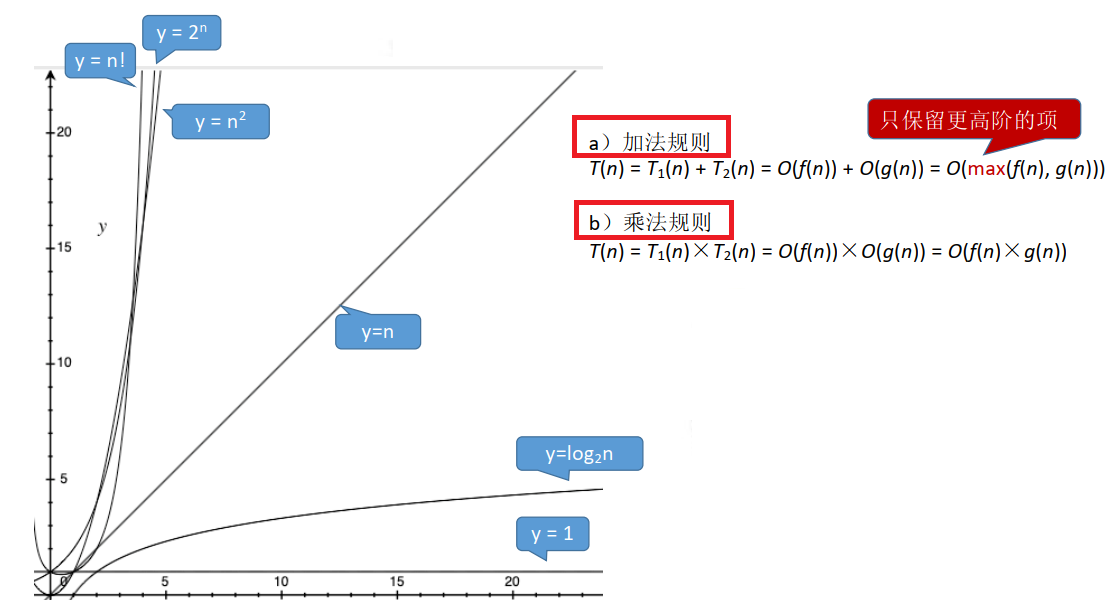#### 例子🌰

1、逐步递增型

① 1次

② 3001次

③④ 3000次

⑤ 1次

T(3000) = 1 + 3001 + 2 * 3000 + 1

T(n) = 3n+3 = O(n)

2、嵌套循环性

$T(n) = O(n) + O(n^2)$ = $O(n^2)$

3、指数递增型

$x = log_2n + 1$

T(n) = O(x) = $O(log_2n)$

4、搜索型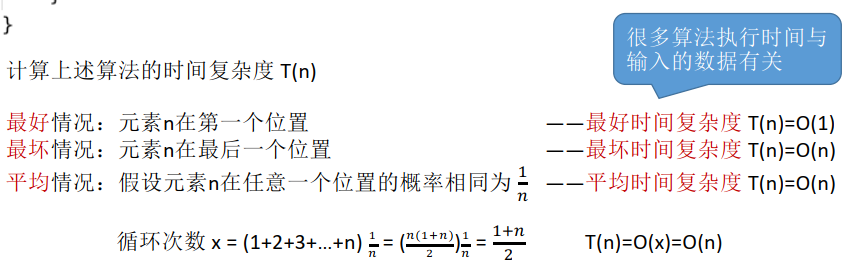### 1.3.3 空间复杂度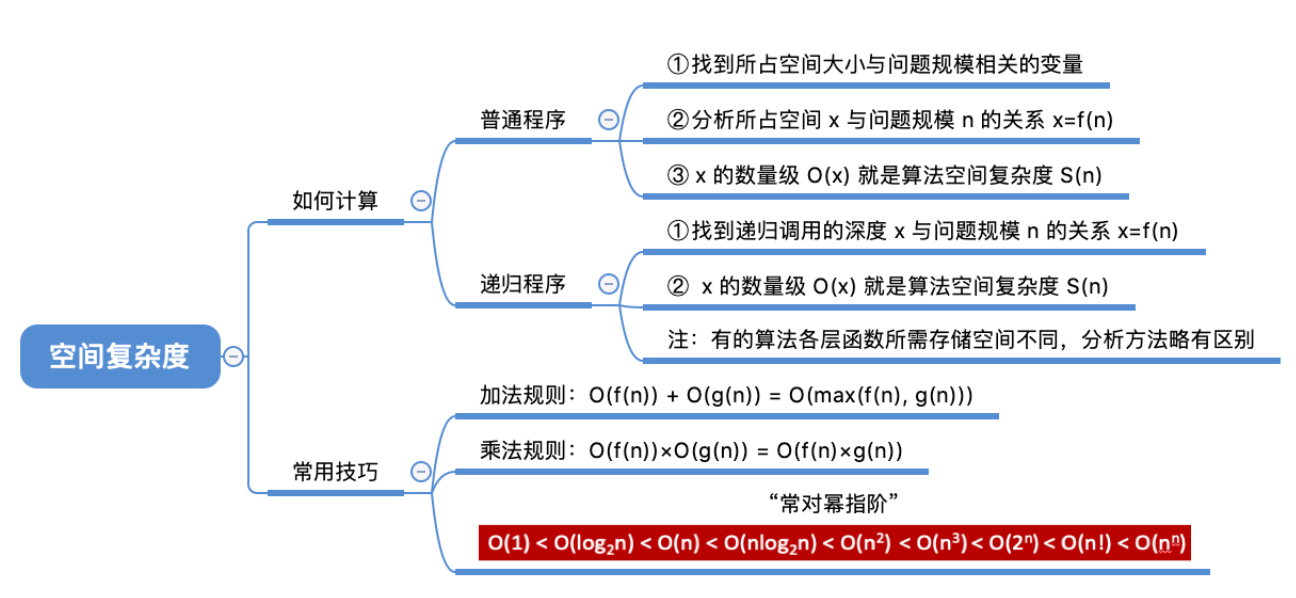（1）逐步递增（2）声明一个一维数组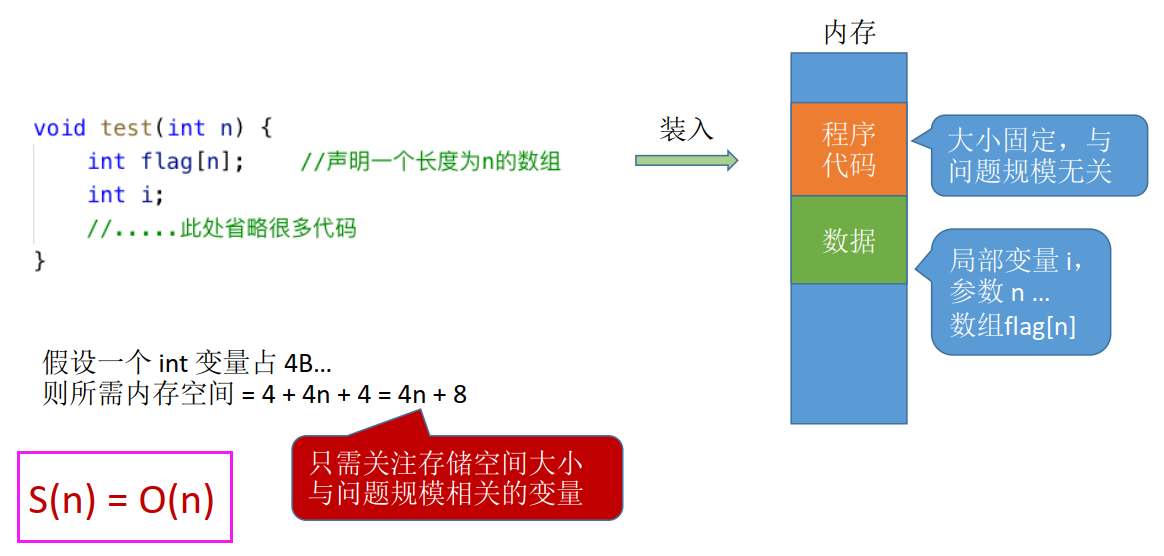（3）声明一个二维数组（4）声明一个二维数组，一个一维数组#### 例子

1、递归型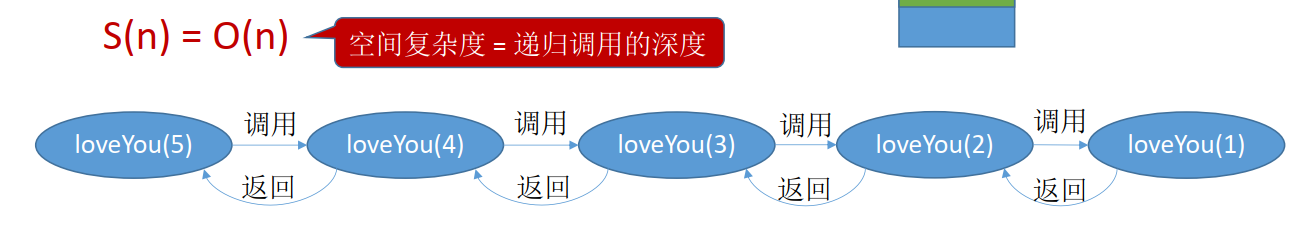2、递归型------ 本文结束感谢您的阅读 ------